# Hydrostatic Pressure Vessel

5364 words (21 pages) Essay in Engineering

31/08/17

Disclaimer: This work has been submitted by a student. This is not an example of the work produced by our Essay Writing Service. You can view samples of our professional work here.

Any opinions, findings, conclusions or recommendations expressed in this material are those of the authors and do not necessarily reflect the views of UK Essays.

Nomenclature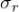= radial stress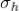= Hoop stress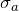= axial stress

Pi = internal pressure

Po = external pressure

ri = internal radius

ro = external radius

r = radius at point of interest (usually ri or ro)

Delta L = Elongation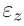= Deformation

ID = inner dimeter of o-ring Gd = groove diameter

Srec = stretch recommended Bd = Bore diameter

GW = Groove width

CS = cross section diameter

C = recommended maximum compression (static)

Summary

A design of a thick vessel is created to measure the effects of hydrostatic pressure when operated in them. The methodology of calculating the stresses on thick walled cylinders is illustrated. A study of the radial and hoop stresses is progressed to find the required sizes of the vessel to operate without a failure under 30 MPa pressure. The bolts are designed to handle the load on the vessel to keep the vessel in one place without moving. The force in the vessel is measured to find the correct bolts that will keep the vessel fixed and safe. A sealing technology study is described to understand how to measure the correct dimension of the o-ring to process the test without air leakage from the entry of vessel. The groove in the vessel was measured to stretch the o-ring designed in a correct manner so the pressure hand move in and out sealed. The calculations in this report are tested mathematically in an academic manner for the design to be brought to life with high efficiency.

Introduction

In the field of science experimenting, it is necessary to test the efficiency of a specific design, because all products of same function have different specifications and differ in quality.

At the same time the true specification of a design can alter its efficiency. Therefore, the best product must be created with real specific measurements that lead to its perfection.

It is found that there are three important features of the pressure vessel. Firstly, the shape of the vessel which presents the equations used to study the impact of pressure in them. Secondly, the material used must be chosen correctly to prevent any danger if vessel fails. Finally, the safety is the most important feature in a pressure vessel hence it is important to choose the correct bolts that will keep the vessel fixed at a point. In addition to designing the desired sealing technology of the vessel to keep the air pressure tight in the vessel without any leakage. Companies, industries and labs usually use hydrostatic pressure on a specimen to figure out it properties which allow us to understand the standards of the material. Using hydrostatic pressure is a safe and effective process which helps us to understand more about failure of objects under pressure and the effect of hydrostatic pressure. There are a few different techniques used to measure the failure of a test, for example, when a hydrostatic test is proceeding it is known that if air starts leaking from the vessel, this would be considered as a failed test.

Thick walled cylinders nature is that they handle more pressure. Under high pressure, the wall might explode and cause failure thus is it important to dimension right size for the thickness of the cylinder before manufacturing the cylinder for hydrostatic testing. Measuring variables such as, hoop, radial, and axial stresses will be discussed and progressed for the vessel designed in this report. The bolts used are entitled to a high load of pressure which is possible to cause a failure if there was no study taken before using these specific bolts that have this mechanical specification. A study on the strength of the bolt is being used and will be illustrated later in the report. Basically, this report will show a study on how to design the required cylinder and bolts to process a safe hydrostatic test. As the design of the vessel will be illustrated to understand the image of the design and its dimensions. Which will lead to an understanding on how to design a specified thick cylinder to process an internal pressure and a hydraulic press to test a specimen hydrostatically.

Aim of the Project

The project aim to design a device to measure the effects of hydrostatic pressure on the fracture toughness of the device. It is found that the device required must handle conditions of 30 MPa pressure thus thick walled cylinder is needed. The importunacy of this project is to create a design that meets the required conditions for it to function plus applying correct methods and calculations for it to function.

Hydrostatic pressure

Hydrostatic pressure test is latest style of testing the ability of fracture toughness of a vessel without causing harm. Hydrostatic test of vessels requires gas or water filled into the vessel if the fracture toughness of vessel is about to crack, the resistance of air reduces the explosion as they resist the inner pressure that causes the failure. Less energy is released when fracture is about to take place LT (2016).

Air is a fair medium that is used for a hydrostatic test. Air is less expensive than oil and it has an easier method than water for a test. The sample will not get harmed after the test. When a failure occurs, it means that the vessel does not meet the standard and this failure can be shown when the durability fails and air begins to leak.

It is important to assure the leak prevention, reliability, and safety of the pressure test. There are two methods of testing hydrostatic and pneumatic. Air is used as a medium of the hydrostatic test Arshad Mahmud (2012).

The hydrostatic test measures the elastic deformation of a metal and its ability to get back to it is formal shape. Air is a safe medium as it is can compressed like water. When a cylinder blows out, air will leak which is safer than using other material due its possibility to be highly explosive.

Pressure vessel

Pressure Vessel is a tank that is designed to contain gases or liquids at different pressure limits. The pressure or temperature drops can be dangerous; this can cause an explosion which can injure anyone near the vessel. Thus, when designing a pressure vessel legal commands must be taken to create a vessel that is safe to perform its task.

History of pressure vessels started in 1495 when Leonardo Da Vinci designed a vessel.

The major problems that occurred were mainly explosions because of leakage of compressed gas or liquid, and if a fire was burning near. Engineers created safety factors as measurements of safety of pressure vessels LT (2016).

Pressure vessels are used in many applications, for example, in compressed air receivers, hot water storage tank, compression chambers, mining operations and nuclear reactor vessels.

A cylinder is the usual shape that is used for hydrostatic testing. It has good maintenance because it is checked every five years for public safety. When the device gets cold when in storage, fatigue might occur hence the importance of the maintenances. However, hard steel responds to it properties to handle in high pressures. Steel also provides controlled and safe environment to prevent any explosion that is possible to harm people.

Shape of the vessel

Cylinders have an ideal shape because it is easy to analyze when in operation and are easily made. This makes cylindrical vessels the cheapest in cost and most effective. Even though the cylinder is the ideal shape for the vessel, it comes with a few disadvantages, for example, the bigger the diameter, the more expensive the construction of the cylinder would be. The ideal size for a cylindrical vessel is 8cm, this is to avoid inspections and testing issues RR (2012). In this case, the maximum internal pressure chosen is 30MPa which allow us to design a thick-walled cylinder. Equation, and variables of thick walled cylinder will be illustrated in this report, based on them a design of a vessel will be created for hydrostatic testing.

Thick Walled Cylinders

There are a lot of examples for thick cylinder such as, guns, hydrostatic testing device, and high pressure hydraulic pipes. Thick cylinders are entitled to internal and external pressure. The wall thickness is large and the stress across the thickness is notable. Stresses in this case are solved by using specific boundary conditions, compatibility, and equilibrium. The problem of thick walled cylinders is that they are entitled to high pressure and temperature which is possible at constant or changing duration. The usual problem is ductile fracture of the material which is because of the geometry or the properties of the material. The real analysis of a thick cylinder is dependent on the radial and hoop stress caused by the internal pressure which stand up to the yield strength of the material.

Equilibrium equation is important because we need it to relate stresses to strains and strains to displacement d(sr) / dr + sr – sh / r = 0.

Compatibility equations;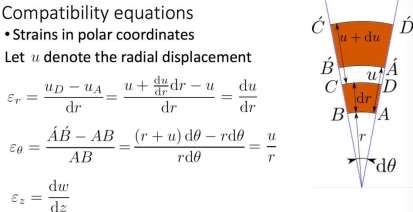Thick walled cylinders have three main mechanical stresses. To design a thick, cylinder the thickness of the wall should be more than 1/10 of the greatest diameter of the cylinder:

1-Hoop Stress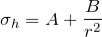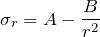3-Axial Stress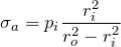(2017) Thick walled cylinder

The boundary conditions of a thick-walled cylinder are:

Thin surface is,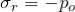at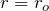and outer surface is,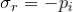at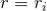Thus -pi = A – (B / ri^2) and -po = A – (B / ro^2)

Variables can be seen in figure 1.1,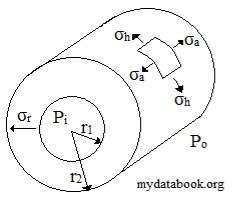Figure 1.1 shows location of the variables (2017) Thick walled cylinder

A and B in the simultaneous equations above when applied to the boundary conditions above, two constant equations for A and B will be concluded;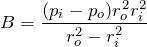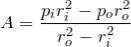Now, to find the final general equation, Lame’s equation is included thus the hoop stress and radial stress will be;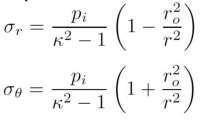(2017) Thick walled cylinder

the axial stress on the case of the cylinder designed bellow which a closed end is calculated by means of equilibrium which is reduced to as shown above.

Maximum shear stress follow that stresses on the cylinder at any point on the wall are principal stresses. Thus, the maximum shear stress at any point will be given by the equation of Tresca theory,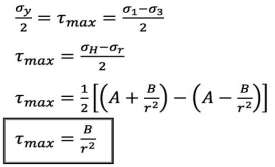Giuseppe Catalanotti (2017)

Material of the vessel

Pressure vessel are mainly made from steel. Rolling or forging is used to make out the shape out of the vessel. Current standards use steel to impact resistance which increases the mechanical strength. Steel is the most important material for engineering and construction in the world. Steel has a great resistance to corrosion because of its formability and durability, high tensile and yield strength and is very effective for thermal conductivity. Stiffness, ductility and yield strength are measured using tensile stress test. Impact test used to figure out the toughness and hardness of surface resistance Total Material (2016).

-Yield strength of steel is 260MPa

-Young modulus is between 210 GPa (Stiffness better three times than aluminum) -density of 7.7/8.1(kg/dm3)

-Poisson’s ratio 0.30

-Thermal conductivity 11.2/48.3(W/mK) -Thermal expansion 9/27(10-6/K)

Total Material (2016).

Safe tank to perform a hydrostatic test must remain sealed. Lip seal technology is used in this system. O-ring were created to prevent any leakage when hydraulic hand is inserted into the chamber. A hydrostatic test should meet these requirements of the vessel to remain testing of pressure safe.

Calculations & Results:

First, the maximum pressure that will cause yield at the internal surface of the vessel will be calculated, using the hoop and radial stresses to find the maximum shear strength and the yield strength. The equations will be used are explained in thick-walled cylinder section which are the hoop and radial stresses. In addition to the maximum shear strength equation and yield equation which will be mentioned in the following;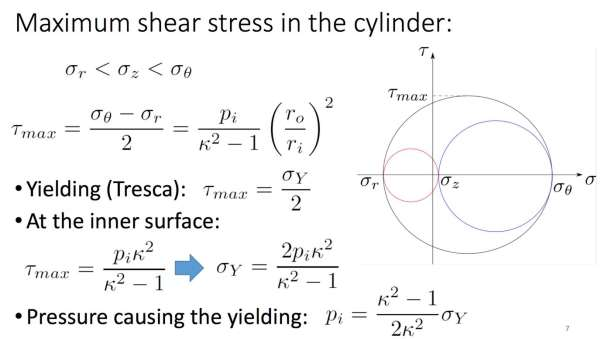Giuseppe Catalanotti (2017)

Secondly, the elongation equation will be used to determine the size of the deformation;

Delta(L) = deformation(z) * L

(L) is the height of the internal cut which has a height of 0.210m Deformation(z)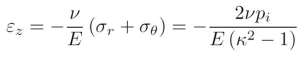v = passion’s ratio

E = the young modulus

k is the ratio of external diameter on the internal diameter ro/ri

First the hoop and radial stresses must be calculated hence the hoop stress equation is

pi = 30MParo = 0.210m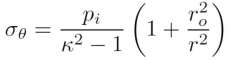k = 2.625ri = 0.08m

hoop stress is = 145.67MPausing hoop stress equation above.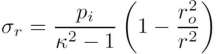pi = 30MParo = 0.210m

k = 2.625ri = 0.08mk = ro / ri

The radial stress is = -(108.75 MPa)but r = -piusing radial stress equation above.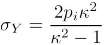The yield equation is

pi = 30*10^6 k = 2.625using the yield equation above.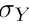= 70.18 MPaSince the yield strength of the steel is 260Mpa and it is greater than the yield at 70.18MPa pressure then the measurements of the vessel appear to be able to handle an extra 190MPa on the system before it begins to fail.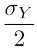The maximum shear strength Tresca isÂ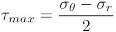Â  =

Since hoop and radial stresses are found, maximum shear stress can be calculated.

= 145.67MPa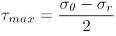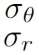= 108.75MPa= 70.18MPa

Giuseppe Catalanotti (2017)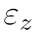Now finding the Elongation which has the equation Delta(L) =* L= (1 – 2v) * pi / E (k^2 – 1)

= (1 – 2 * 0.3) * 30*10^6 / 210*10^9 * (2.625^2 – 1) = 9.7*10^-6

v = passion’s ratio of steel pi = internal pressure

E = the young modulus of steel

k is the ratio of external diameter on the internal diameter ro/ri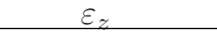Deformation= 9.7 *10^-6m

Since, Delta(L) = defamation(z) * L

Then,

L = Length of the cylinder which is = 0.21m. See figure (3).

 Elongation is 9.7 * ^-6 * 0.21 = 2.037 *10^-6m from Elongation equation above Giuseppe Catalanotti (2017)

Bill of Materials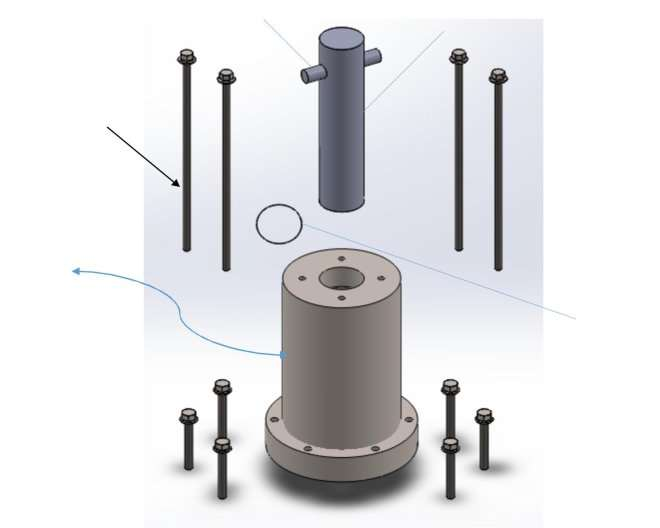(6)

(4)

(1)

(3)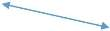(5)

(2)

 Item Number Description quantity Material (1) Vessel 1 Steel (2) O-Ring 1 Rubber (3) M14 Bolts 6 Steel (4) M12 Bolts 4 Steel (5) Pressure hand 1 Steel (6) Holding Stick 1 SteelThe Design and assembly procedure

A hydrostatic pressure device was designed to measure the pressure. This tank is to be attached to the hydraulic machine. Measurement of the machine were taken to design the vessel. The purpose of this device in figure (1) is to ensure that pressure tests are maintained safely and properly. This design is measured to handle pressure between 0MPa to 70MPa. The test will be progressed at 30 MPa. The material used is steel.

The design contains six different main parts as shown in figure (1). This section will provide dimension and function of each part.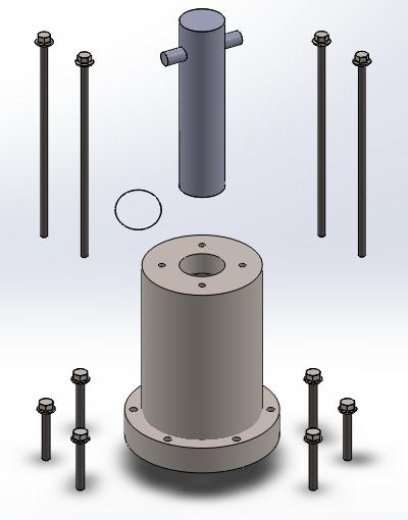Figure (1) Vessel Components

(Exploded View)

The size and the thickness are very important factors when designing a safe vessel. Figure (2) shows the circular diameter of the head of the vessel which is 21cm and that it was extruded to 34cm. Figure (2) is also a cross sectional view of the final shape of the vessel.The thichness of the wall surrounding the chamber is 6.5cm and the bottom has a thickness of 7cm.

Figure (3) shows the dimsion of the chamber which is a cylinder of 8cm diameter and length of 21cm. This area is where it is expected to place the specimen. The specimen size is measured to be 20*50*4mm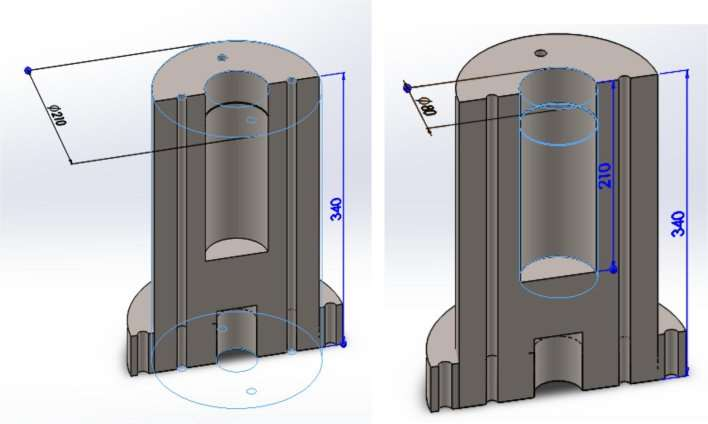Figure (2) Dimension (1)Figure (3)Chamber dimensions

The mechanism that will hold the vessel is smart. Figure (4) shows a diameter of a cut of 5.1 cm and 6.5 high. This area is meant to be placed into the bottom holding hand of the hydraulic machine in the Ashby building. Where the vessel has an extrusion of 27cm and height of 5cm and was created as a base of vessel as shown in figure (5).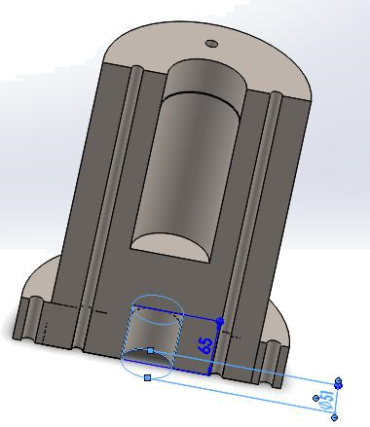Figure (4) Bottom hand holder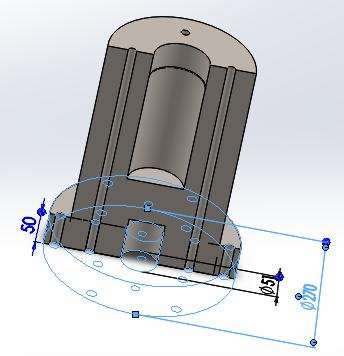Figure (5) shows the base diameter and height

Figure (6) shows that the base cylinder has 6 holes of M14 size. These holes were created to be tighten up with bolts of M14 shown in figure (7) on the table of the hydraulic machine. Six bolts are created and can be seen in figure (6).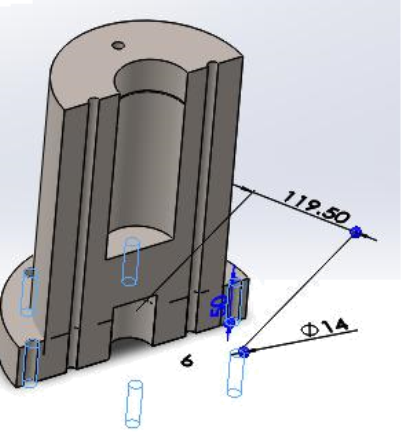Figure-(6) M14 holes x6

Specification of the Bolts

(F = P x A),which means that the force is equal to the pressure multiplied by the area. Figure

(3) shows the internal diameter of the vessel which is 0.08m. (a = pi x (d)^2 / 4) is used to calculate the area of a cylinder, which means that (pi x (0.08m)^2 / 4) = (50.03*10^-3 m^2).

The maximum pressure will be tested is 30 MPa. Since pressure is 30MPa and area is

(5.03*10^-3 mm^2). then as they multiplied to bring a force of 150 kN. This means that thetotal force in the cylinder is equal to 150 kN.

The question here is that will the bolts in figure (7) and (8) M14, M12 handle 150 kN of force. The research on the metric bolts shows the ability of each size of the bolts. It was shown that M14 bolt has a load proof of 66.7 kN. Figure (6) shows that there are 6 bolts of M14 is used. Now, the total force applied is divided by the number of the bolts to show the required ability of the bolts. (150 kN / 6 = 25 kN) which means that the system requires six bolts that each of them can handle 25 kN of force Metric Bolts (2016).

Above it is mentioned that the load proof of each of the M14 bolts is 66.7 kN which means that a total of six M14 bolts will provide an ability of 400 kN, 400 kN of force can handle 150 kN of applied force. In brief, the bolts used in the system in figure (11) meet the requirement of the study which is 30 MPa.

To ensure the safety of fixing the vessel to the machine, four M12 bolts were added. M12 bolt figure (8) has a load proof of 48.9 kN, Total load proof of four bolts is equal to 195.6 kN which has a good impact on keeping the vessel safe from any danger of being rapidly moved away from it fixed point. Eventually, the total ability of the bolts is equal to 595.6 kN which are designed to prevent the vessel from any possible danger of a maximum pressure load of 150 kNMetric Bolts (2016).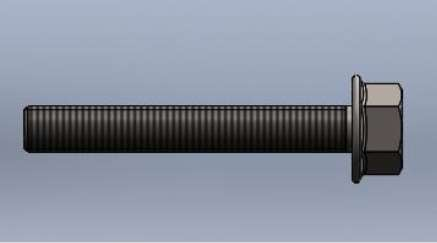Figure (7) M14 Bolt x6

Figure (1) shows 4 holes created on top of the vessel. They are M12 holes created to increase stability of the vessel and more safety in case of the vessel left it place. Bolts of M12 were created to have a length of 39cm as shown figure (8).

Calculations above are measurements of parts in figure (1) . Ten bolts, 4 of M12 and 6 of M14 are attached to the base of hydraulic machine. Finally, a hydrostatic test can be done safely.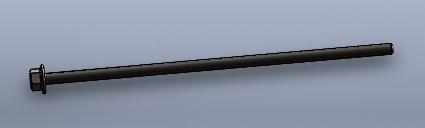Figure (8) Long M12 bolts x4

Sealing Technology

O-ring is a mechanical gasket in a shape of loop with a diameter that is made of rubber. It is designed to be fitted at circler cut which means that an o-ring will be compressed during work. It is expected to seal a joint of two parts. One mechanical benefit of the o-rings is to seal a moving hand through a vessel without an air leakage. Thus, a specific design of an o-ring will be designed to be fitted at the entry gate of the vessel for the hand to enter the vessel without air leaking (efunda) 2017.

Figure (5) shows the place of the o-ring. The thickness of the o-ring is calculated to be 0.84 mm and the inner diameter of the o-ring is measured to be 79.46 mm. The o-ring designed can be seen in figure (10).

Figure (9) shows the grave and the dimension of the O-ring. An o-ring is meant to be attached in this system. The groove was measured to be 4 cm away from the top of the vessel in the chamber section. The groove diameter was calculated to be 8.1 cm and the width of the groove is 1.15 mm.

As hydraulic hand enters the chamber, it will pass through three rubber bands that reduces the area of the chamber making it tighter for the hand to be inserted without any leakage outside the system P&S (2016).

The design of the grave and the o-ring must be studied before they are designed. To design a sealing technology there are a few variables to know to create it. These variables are the bore dimeter of the outside radius, the groove diameter of the inside radius and the groove width of the axial length. The maximum stretch of the 0-ring should not be more than 5% of the grove size (efunda) 2017.

Calculations of the O-ring and Results

ID is the inner diameterof the o-ring and can be found if Srec the recommended stretchischosen and the groove diameter Gd the groove diameter can also be found when this equation

is used;

Srec = 2%

ID = Gd * (1 – Srec) then, ID = Gd * (0.98). The stretch of the o-ring is to make the o-ringstay at the groove tightly without falling out (efunda).

ID = (39.94 * 2) – 0.42 = 79.46 mm

ID = 79.46mm

Gd = ID / 0.98

Gd = 79.46 / 0.98 = 81mm

Gd = 81mm

Cross sectional Diameter (CS) of the o-ring:

O-ring will be compressed in radial direction when it is attached to the groove. As the cross section of the ring is compressed between groove and bore diameter then the cross-sectional diameter must be more the depth of the groove. Which means that CS > Gd – ID / 2 (efunda) 2017.

CS = Gd – ID / 2 =

81mm- 79.46mm / 2 = 0.77mm

As CS must be > than 0.77mm then CS = 1mm

C is the recommended maximum compression is 40% and must be for the ring to be compressed. The recommendation of C depended on the seal kind. Static seals do not require an axial movement in the bore(efunda) 2017.

Groove Width in the Vessel (GW) =

When the ring is compressed horizontally it will expand vertically. The material of the ring will be helpful to be compressed effectively. However, the groove width should be about 1.5 by the cross sectional of the o-ring diameter and this to stretch the ring vertically (efunda) 2017.

GW = CS * (1.5) = 1mm * 1.5mm = 1.5mm.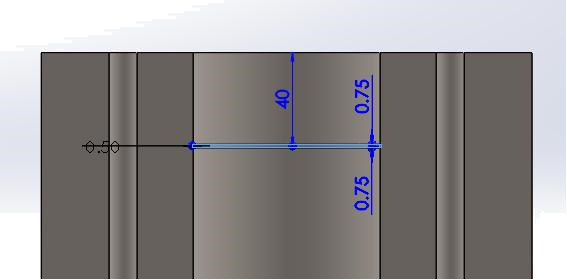Figure (9) Section Cut shows the position and size of the Groove width and diameter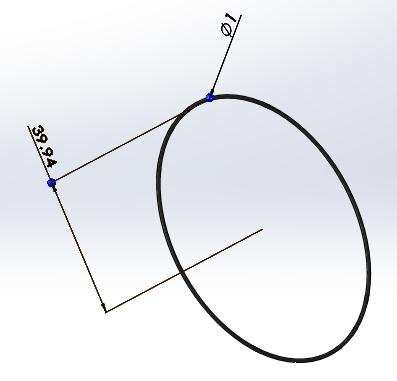Figure (10) O-ring CS dimeter and radius (r)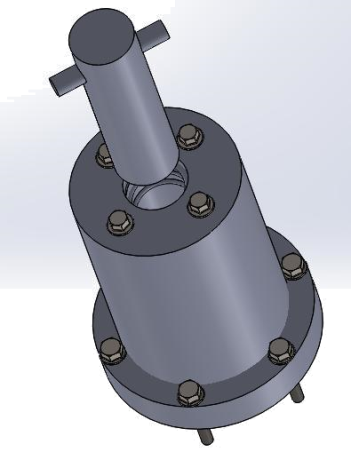Figure (11) Final Product (Thick walled Vessel)

Discussion

Thick Walled cylinders handle high pressures with least effects on the vessel and remain safe for future use. The deformation possible in the vessel is calculated to find the amount of elongation (the change in formation or length in an object). The elongation value is 0.002mm which is proper. The maximum pressure in the vessel is 30MPa hence finding the hoop and radial stresses to find the shear stress of the vessel and compare it with the yield strength of the vessel’s material and it dimensions. Therefore, the stresses applied at different sections in the chamber are calculated above and hoop stress is found to be 145.67MPa and radial stress is

108.7MPa. The maximum shear stressin the vessel is calculated from the hoop and radialstresses and it is found to be 70.18 MPa. Based on Total Material (2016) the yield strength of steel is 260 MPa. Giuseppe Catalanotti (2017) have stated that if yield strength of material is bigger than the shear stress calculated in the vessel then the vessel is safe when pressure applied. The Bolts are designed to handle keep the vessel fixed on the ground of the hydraulic machine. The total force initiated in the vessel is calculated and found to be 150kN thus the required bolts are must have a higher force than 150kN. The six M14 bolts used can handle force of 400kN and this enough for the vessel to function safely. Finally, the sealing technology, the o-Â­ring is designed to keep the vessel sealed when it is preforming. (efunda) 2017 stated that the maximum recommended stretch of an o-Â­ring is 5% and the designed o-Â­ring has a stretch of 2%. The groove width is 8.1cm, calculated using 2% max. stretch and an inner diameter of 7.94cm. The CS diameter o-Â­ring is 1mm, which must be higher than the calculatedCS diameter which is 0.77mm. Hence, the

#### Cite This Work

To export a reference to this article please select a referencing stye below:

## Related Services

View all

### DMCA / Removal Request

If you are the original writer of this essay and no longer wish to have the essay published on the UK Essays website then please.Prices from
###### £28

Undergraduate 2:2 • 250 words • 7 day delivery

Delivered on-time or your money back

##### Rated 4.6 out of 5 by(69 Reviews)

## Our Services

### Resources

Untitled Document